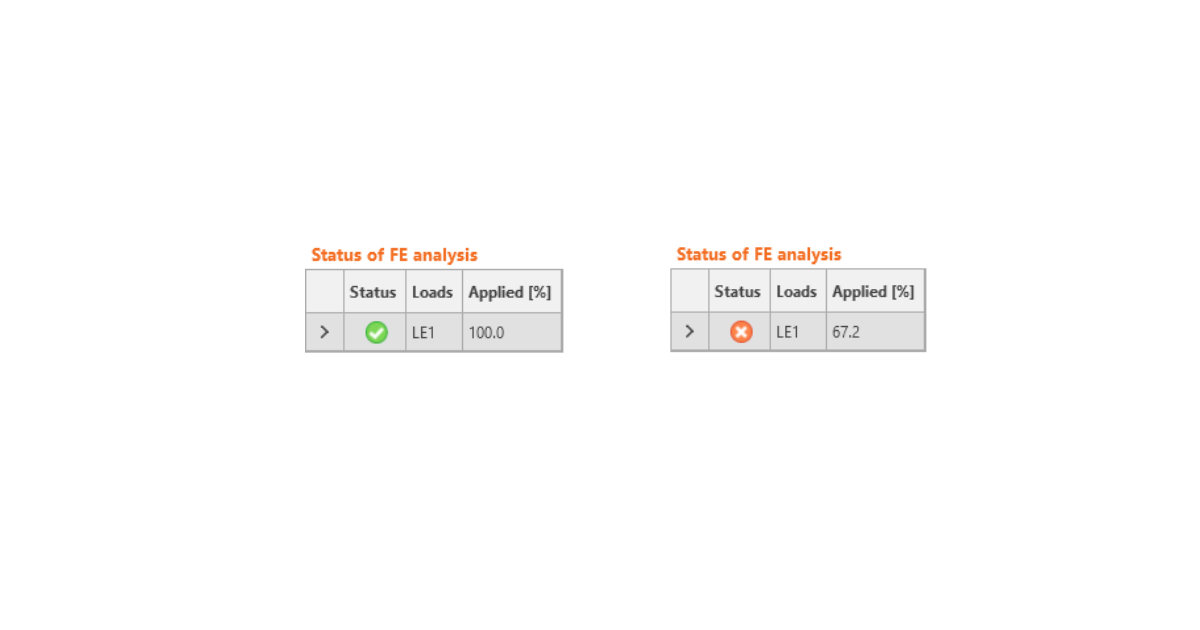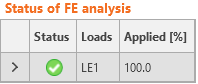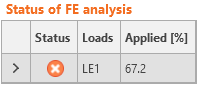### Válasszon nyelvet,# Strength analysis of steel joints

SupportCenter.LocalizedArticle
$$Strength analysis is the most important analysis of joints. Strain checks of plates together with code checks of components are performed by elastic-plastic analysis.The analysis of joints is materially non-linear. The load increments are applied gradually, and the state of stress is searched. There are two optional analysis modes in IDEA StatiCa Connection: The response of structure (joint) to the overall load. All defined load (100 %) is applied in this mode, and the corresponding state of stress and deformation is calculated.Analysis termination at reaching the ultimate limit state. The checkbox in Code setup “Stop at limit strain” should be ticked. The state is found when the plastic strain reaches the defined limit. In the case when the defined load is higher than the calculated capacity, the analysis is marked as non-satisfying, and the percentage of used load is printed. Note that the analytical resistance of components, for example of bolts, can be exceeded.The second mode is more suitable for practical design. The first one is preferable for a detailed analysis of complex joints.$$